# Selina Solutions Concise Maths Class 10 Chapter 7 Ratio and Proportion Exercise 7(A)

In this exercise, the concept of ratio, increase (or decrease) in a ratio, commensurable and incommensurable quantities and composition of ratios are majorly tested. For any further clarifications regarding the method of solving problems, the Selina Solutions for Class 10 Maths can be used by students. Additionally, the solutions to this exercise are available in the Concise Selina Solutions for Class 10 Maths Chapter 7 Ratio and Proportion Exercise 7(A) PDF in the links given below.

## Selina Solutions Concise Maths Class 10 Chapter 7 Ratio and Proportion Exercise 7(A) Download PDF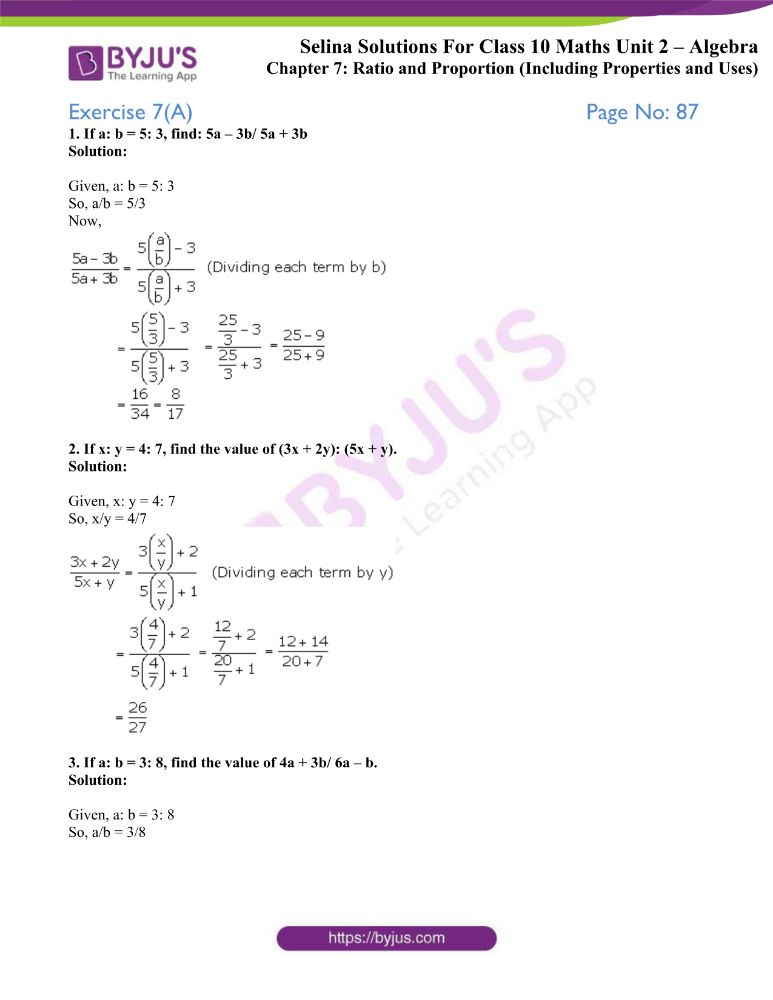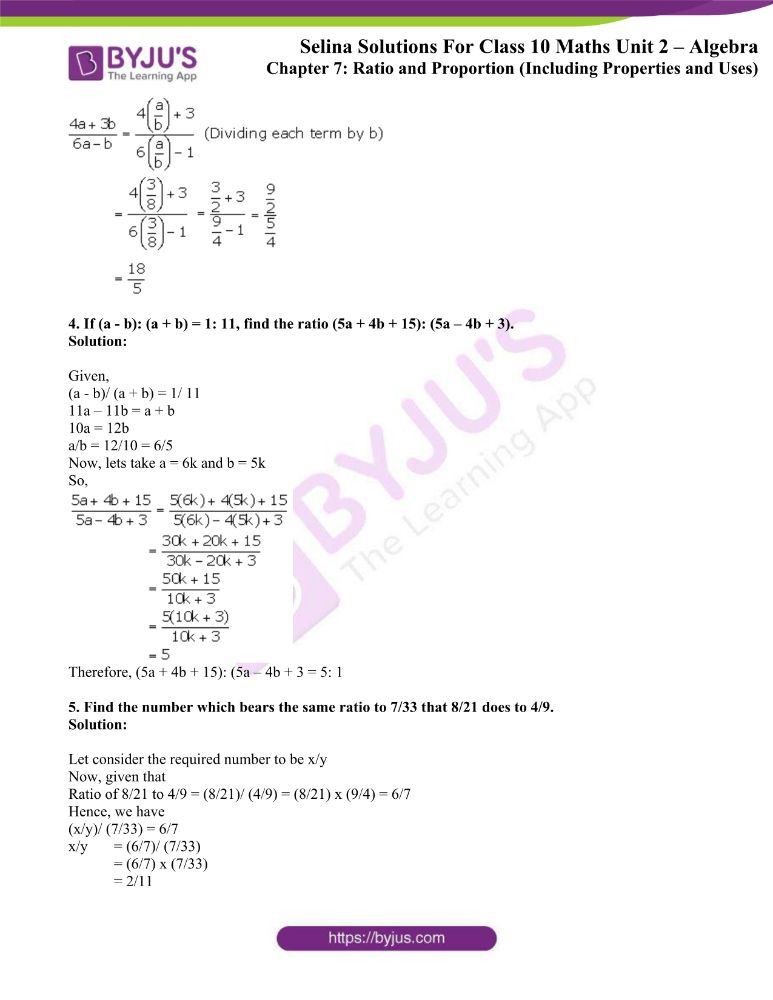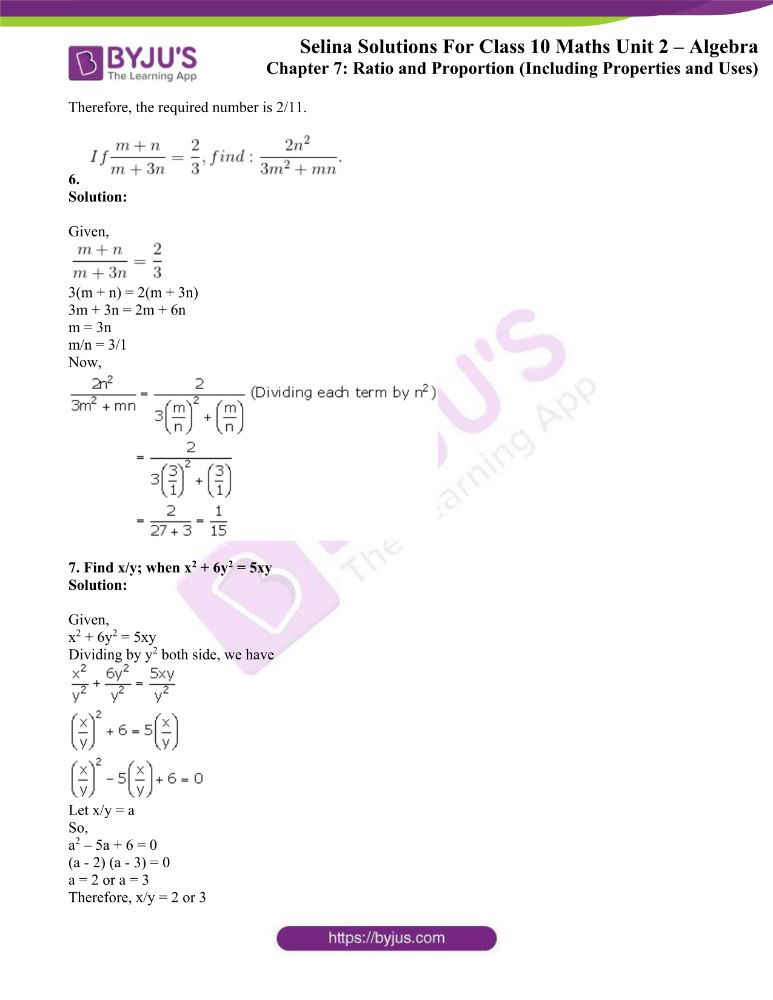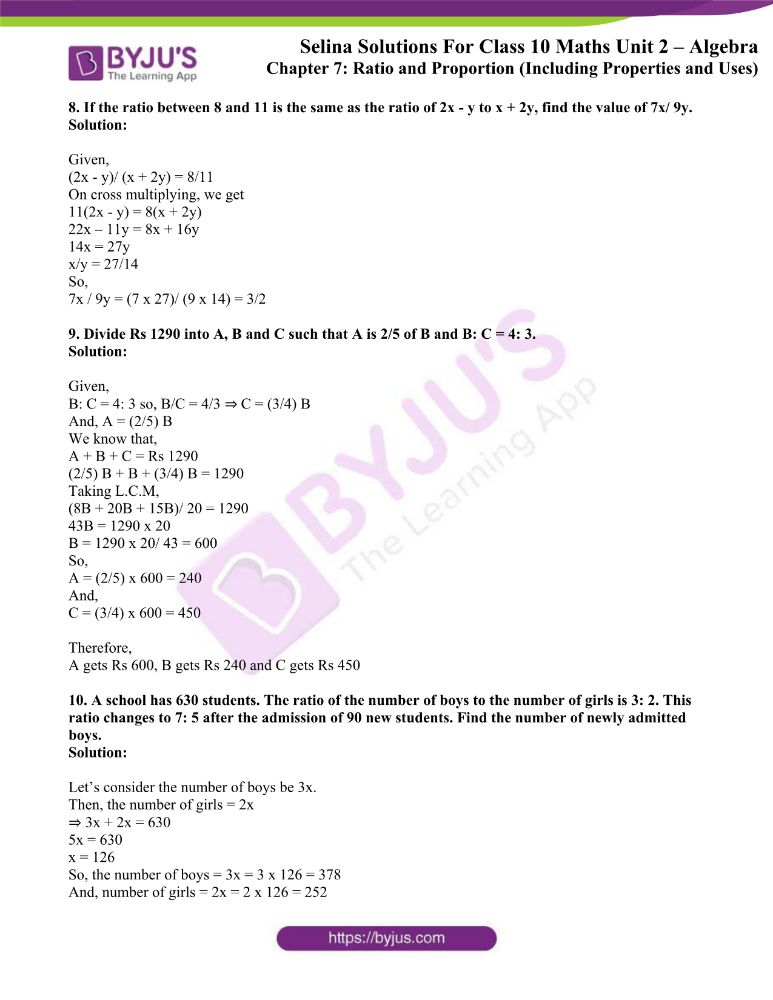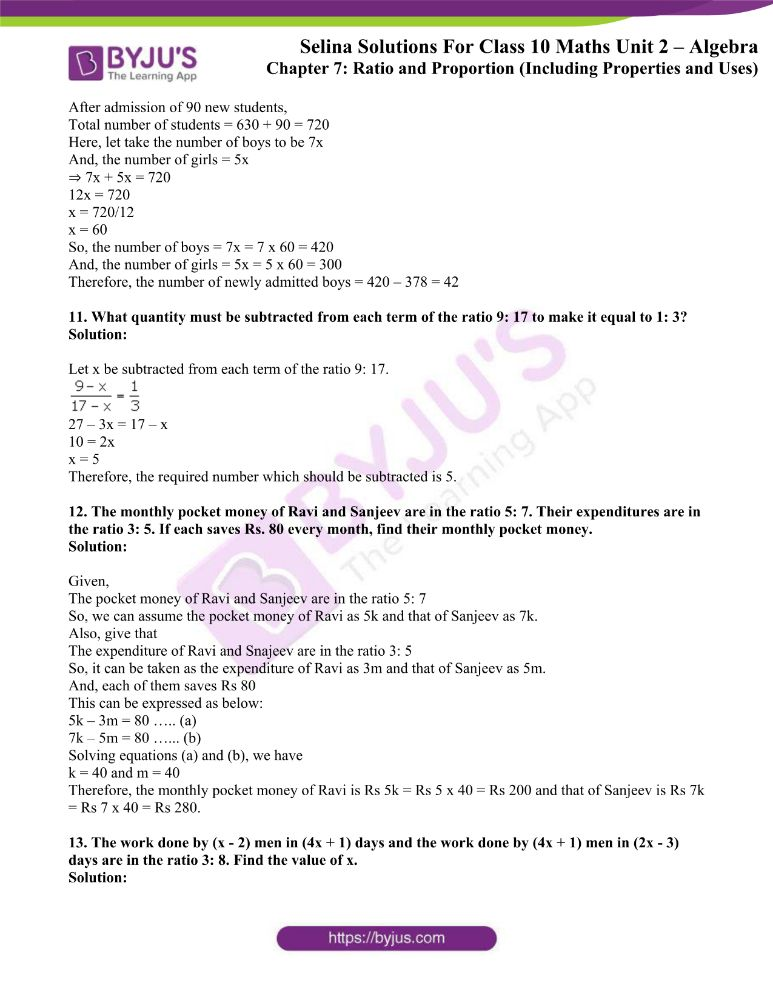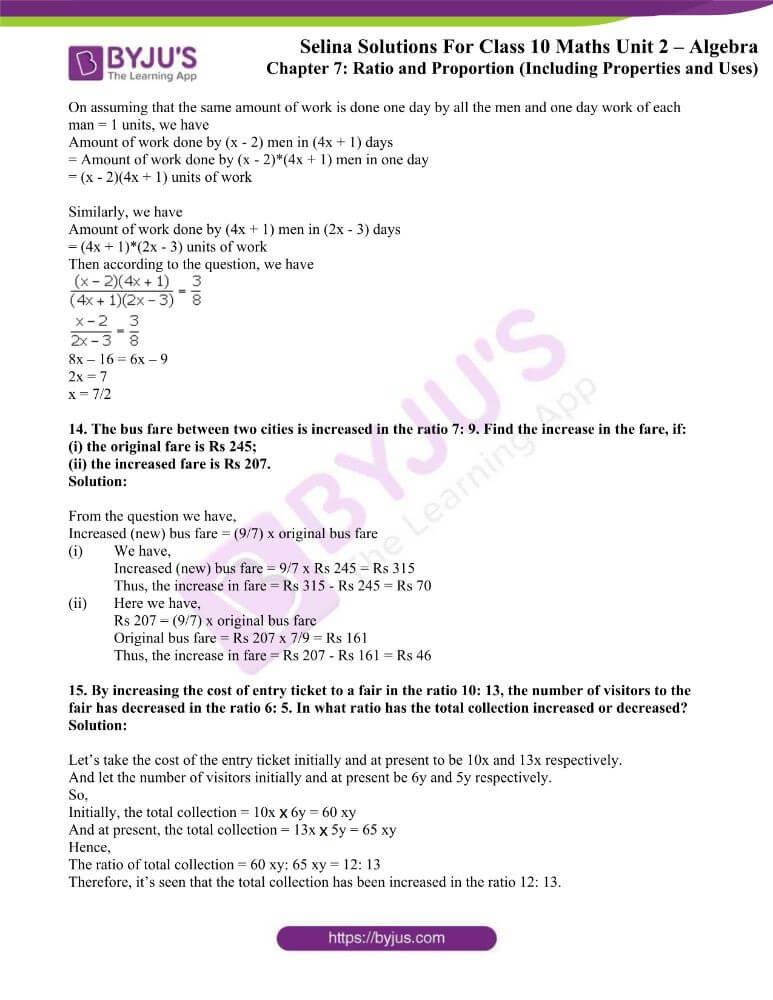### Access other exercises of Selina Solutions Concise Maths Class 10 Chapter 7 Ratio and Proportion

Exercise 7(B) Solutions

Exercise 7(C) Solutions

Exercise 7(D) Solutions

### Access Selina Solutions Concise Maths Class 10 Chapter 7 Ratio and Proportion Exercise 7(A)

1. If a: b = 5: 3, find: 5a – 3b/ 5a + 3b

Solution:

Given, a: b = 5: 3

So, a/b = 5/3

Now,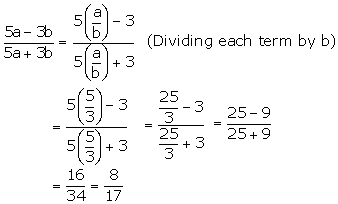2. If x: y = 4: 7, find the value of (3x + 2y): (5x + y).

Solution:

Given, x: y = 4: 7

So, x/y = 4/7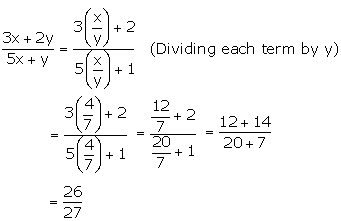3. If a: b = 3: 8, find the value of 4a + 3b/ 6a – b.

Solution:

Given, a: b = 3: 8

So, a/b = 3/8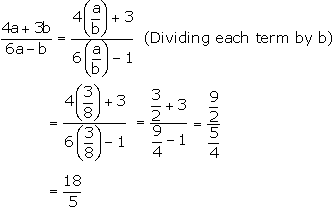4. If (a – b): (a + b) = 1: 11, find the ratio (5a + 4b + 15): (5a – 4b + 3).

Solution:

Given,

(a – b)/ (a + b) = 1/ 11

11a – 11b = a + b

10a = 12b

a/b = 12/10 = 6/5

Now, lets take a = 6k and b = 5k

So,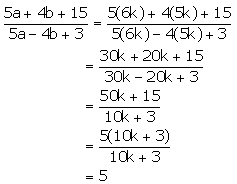Therefore, (5a + 4b + 15): (5a – 4b + 3 = 5: 1

5. Find the number which bears the same ratio to 7/33 that 8/21 does to 4/9.

Solution:

Let consider the required number to be x/y

Now, given that

Ratio of 8/21 to 4/9 = (8/21)/ (4/9) = (8/21) x (9/4) = 6/7

Hence, we have

(x/y)/ (7/33) = 6/7

x/y = (6/7)/ (7/33)

= (6/7) x (7/33)

= 2/11

Therefore, the required number is 2/11.

6.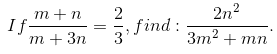Solution:

Given,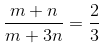3(m + n) = 2(m + 3n)

3m + 3n = 2m + 6n

m = 3n

m/n = 3/1

Now,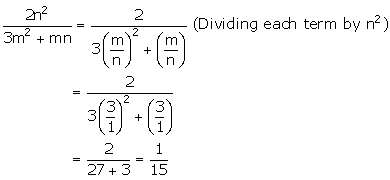7. Find x/y; when x2 + 6y2 = 5xy

Solution:

Given,

x2 + 6y2 = 5xy

Dividing by y2 both side, we have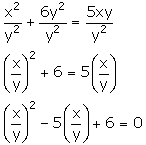Let x/y = a

So,

a2 – 5a + 6 = 0

(a – 2) (a – 3) = 0

a = 2 or a = 3

Therefore, x/y = 2 or 3

8. If the ratio between 8 and 11 is the same as the ratio of 2x – y to x + 2y, find the value of 7x/ 9y.

Solution:

Given,

(2x – y)/ (x + 2y) = 8/11

On cross multiplying, we get

11(2x – y) = 8(x + 2y)

22x – 11y = 8x + 16y

14x = 27y

x/y = 27/14

So,

7x / 9y = (7 x 27)/ (9 x 14) = 3/2

9. Divide Rs 1290 into A, B and C such that A is 2/5 of B and B: C = 4: 3.

Solution:

Given,

B: C = 4: 3 so, B/C = 4/3 ⇒ C = (3/4) B

And, A = (2/5) B

We know that,

A + B + C = Rs 1290

(2/5) B + B + (3/4) B = 1290

Taking L.C.M,

(8B + 20B + 15B)/ 20 = 1290

43B = 1290 x 20

B = 1290 x 20/ 43 = 600

So,

A = (2/5) x 600 = 240

And,

C = (3/4) x 600 = 450

Therefore,

A gets Rs 600, B gets Rs 240 and C gets Rs 450

10. A school has 630 students. The ratio of the number of boys to the number of girls is 3: 2. This ratio changes to 7: 5 after the admission of 90 new students. Find the number of newly admitted boys.

Solution:

Let’s consider the number of boys be 3x.

Then, the number of girls = 2x

⇒ 3x + 2x = 630

5x = 630

x = 126

So, the number of boys = 3x = 3 x 126 = 378

And, number of girls = 2x = 2 x 126 = 252

After admission of 90 new students,

Total number of students = 630 + 90 = 720

Here, let take the number of boys to be 7x

And, the number of girls = 5x

⇒ 7x + 5x = 720

12x = 720

x = 720/12

x = 60

So, the number of boys = 7x = 7 x 60 = 420

And, the number of girls = 5x = 5 x 60 = 300

Therefore, the number of newly admitted boys = 420 – 378 = 42

11. What quantity must be subtracted from each term of the ratio 9: 17 to make it equal to 1: 3?

Solution:

Let x be subtracted from each term of the ratio 9: 17.27 – 3x = 17 – x

10 = 2x

x = 5

Therefore, the required number which should be subtracted is 5.

12. The monthly pocket money of Ravi and Sanjeev are in the ratio 5: 7. Their expenditures are in the ratio 3: 5. If each saves Rs. 80 every month, find their monthly pocket money.

Solution:

Given,

The pocket money of Ravi and Sanjeev are in the ratio 5: 7

So, we can assume the pocket money of Ravi as 5k and that of Sanjeev as 7k.

Also, give that

The expenditure of Ravi and Snajeev are in the ratio 3: 5

So, it can be taken as the expenditure of Ravi as 3m and that of Sanjeev as 5m.

And, each of them saves Rs 80

This can be expressed as below:

5k – 3m = 80 ….. (a)

7k – 5m = 80 …… (b)

Solving equations (a) and (b), we have

k = 40 and m = 40

Therefore, the monthly pocket money of Ravi is Rs 5k = Rs 5 x 40 = Rs 200 and that of Sanjeev is Rs 7k = Rs 7 x 40 = Rs 280.

13. The work done by (x – 2) men in (4x + 1) days and the work done by (4x + 1) men in (2x – 3) days are in the ratio 3: 8. Find the value of x.

Solution:

On assuming that the same amount of work is done one day by all the men and one day work of each man = 1 units, we have

Amount of work done by (x – 2) men in (4x + 1) days

= Amount of work done by (x – 2)*(4x + 1) men in one day

= (x – 2)(4x + 1) units of work

Similarly, we have

Amount of work done by (4x + 1) men in (2x – 3) days

= (4x + 1)*(2x – 3) units of work

Then according to the question, we have8x – 16 = 6x – 9

2x = 7

x = 7/2

14. The bus fare between two cities is increased in the ratio 7: 9. Find the increase in the fare, if:

(i) the original fare is Rs 245;

(ii) the increased fare is Rs 207.

Solution:

From the question we have,

Increased (new) bus fare = (9/7) x original bus fare

(i) We have,

Increased (new) bus fare = 9/7 x Rs 245 = Rs 315

Thus, the increase in fare = Rs 315 – Rs 245 = Rs 70

(ii) Here we have,

Rs 207 = (9/7) x original bus fare

Original bus fare = Rs 207 x 7/9 = Rs 161

Thus, the increase in fare = Rs 207 – Rs 161 = Rs 46

15. By increasing the cost of entry ticket to a fair in the ratio 10: 13, the number of visitors to the fair has decreased in the ratio 6: 5. In what ratio has the total collection increased or decreased?

Solution:

Let’s take the cost of the entry ticket initially and at present to be 10x and 13x respectively.

And let the number of visitors initially and at present be 6y and 5y respectively.

So,

Initially, the total collection = 10x x 6y = 60 xy

And at present, the total collection = 13x x 5y = 65 xy

Hence,

The ratio of total collection = 60 xy: 65 xy = 12: 13

Therefore, it’s seen that the total collection has been increased in the ratio 12: 13.